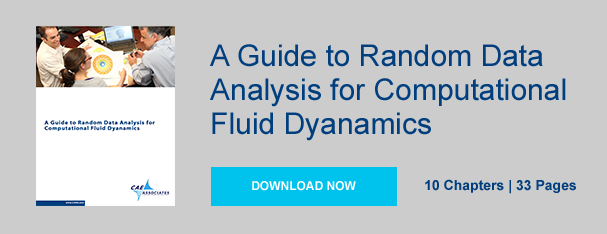you are here:/ News & Insights / Engineering Advantage Blog / Random Data Analysis Part 4: Application of The Fast Fourier Transform

# Random Data Analysis Part 4: Application of The Fast Fourier TransformOctober 16, 2015 By: Jonathan Dudley

In my previous post, I discussed the required inputs when performing a Fast Fourier Transform (FFT) analysis.  For this blog, I would like to execute the FFT for a representative data set and discuss the effects of the chosen parameters. Again, I will focus this talk around the built in function in Python (Anaconda) to generate the FFT’s using Welch’s method using the call given below:

Pxx,f=mpl.psd(pfluc,nfft,fs,window=mlab.window_hanning,scale_by_freq=
False,sides='onesided',noverlap=nlap).

Selection of nfft:

Based on the frequency resolution given by Equation 1 it is easy to see that the larger the size of your nfft the more accurate your frequency resolution becomes for a fixed sampling rate.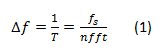Unfortunately, by increasing your nfft you lower the number of available periodograms used in the averaging of the final FFT, as each window has now been extended. To mitigate this, you can use overlapping. Typically, the optimal overlap percentage is between 50 and 75% of the nfft size. Ultimately, the choice of nfft becomes a balancing act between the desired accuracy of the frequency resolution vs. the magnitude of the spectra.

Let’s assume we have a cavity that resonates at the lowest frequency near 1 [kHz] as presented in the last post. Let’s further assume we have the capability for infinite sampling frequency. In this scenario, the lowest frequencies are the most demanding to capture because they require they longest sampling times. For our scenario, the sampling frequency is fixed at 1e-05 [s] or 100 [kHz] with N=219 total points. We desire at least 20 cycles per nfft period to ensure statistical accuracy (the more the better, of course). This means we would need at least 0.05 [s] of simulation time and 5,000 points per nfft. We have now given ourselves a reasonable estimate for our selection of nfft that should be between 212 and 213. The trade-off here is with the accuracy of the resulting frequency resolution as seen in Table 1. But, we also want to average multiple periodograms (per Welch’s method) to ensure accuracy. Thus, we may want on the order of 30 ensembles which requires at least a total signal length of N=150,000 points. Remember, we are using powers of 2 so we really need the next power of 2, which would be 218.

Table 1. below illustrates our corresponding frequency resolution (bin width), number of averaged periodograms, and the number of shedding cycles per period for selected values of nfft.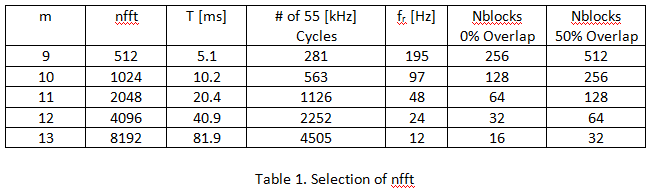The following figures were generated using the FFT call presented at the top of this post with a Hanning window and no overlapping. Figure 1 illustrates the effect of the selection of nfft on your FFT. It is evident that as the period was shortened (consequently the frequency resolution is higher), that the peak values decrease. Further, there is substantial broadening of the peaks. This is indicative of the power being dispersed as a consequence of wider frequency bins.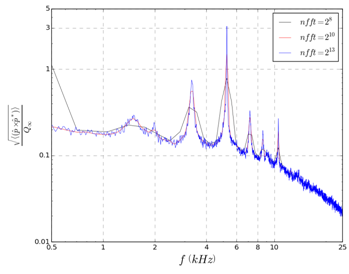Figure 2 illustrates the effect of the selection of frequency resolution on your FFT. The original signal length was decimated by a factor of 2 and 4 to emulate larger sampling frequencies. Similar to Figure 1, the peak values decrease and the broadening of the peaks is still evident as the frequency resolution grows.

This brings to light that when comparing experimental spectral plots with those computed from a CFD analysis, that care must be taken to understand exactly how the plots were constructed (not just the physics of the problem!). It is important that the spectral plots be constructed in the same fashion. If complete information is not supplied on how the FFT was performed, one may end up with misleading discrepancies when comparing back to back figures!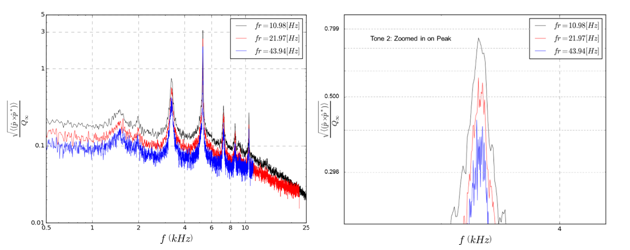Please watch for Part 5 of this series where I will discuss a simple method for checking the accuracy of your FFT. If you have further experiences or questions please feel free to comment!